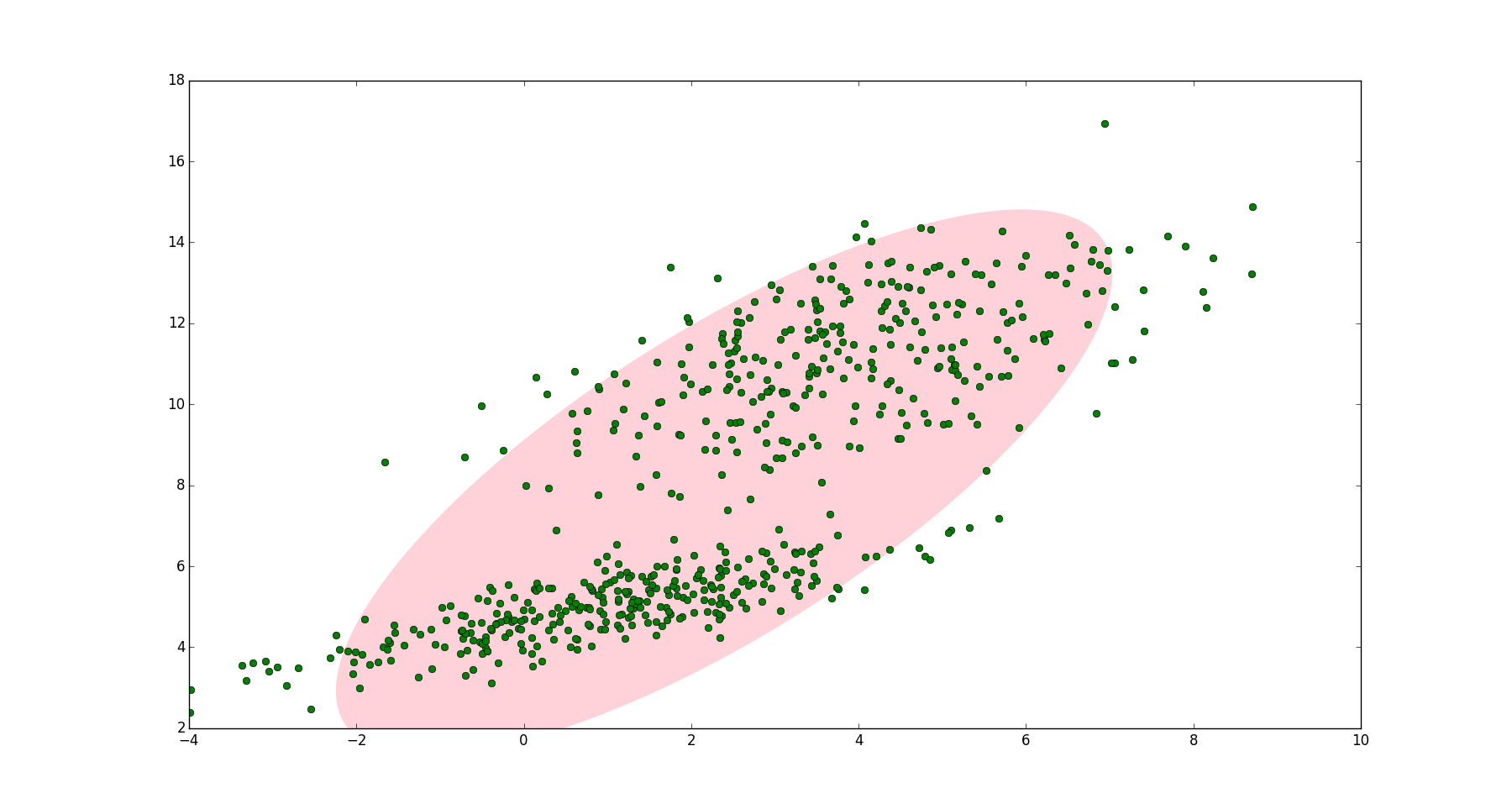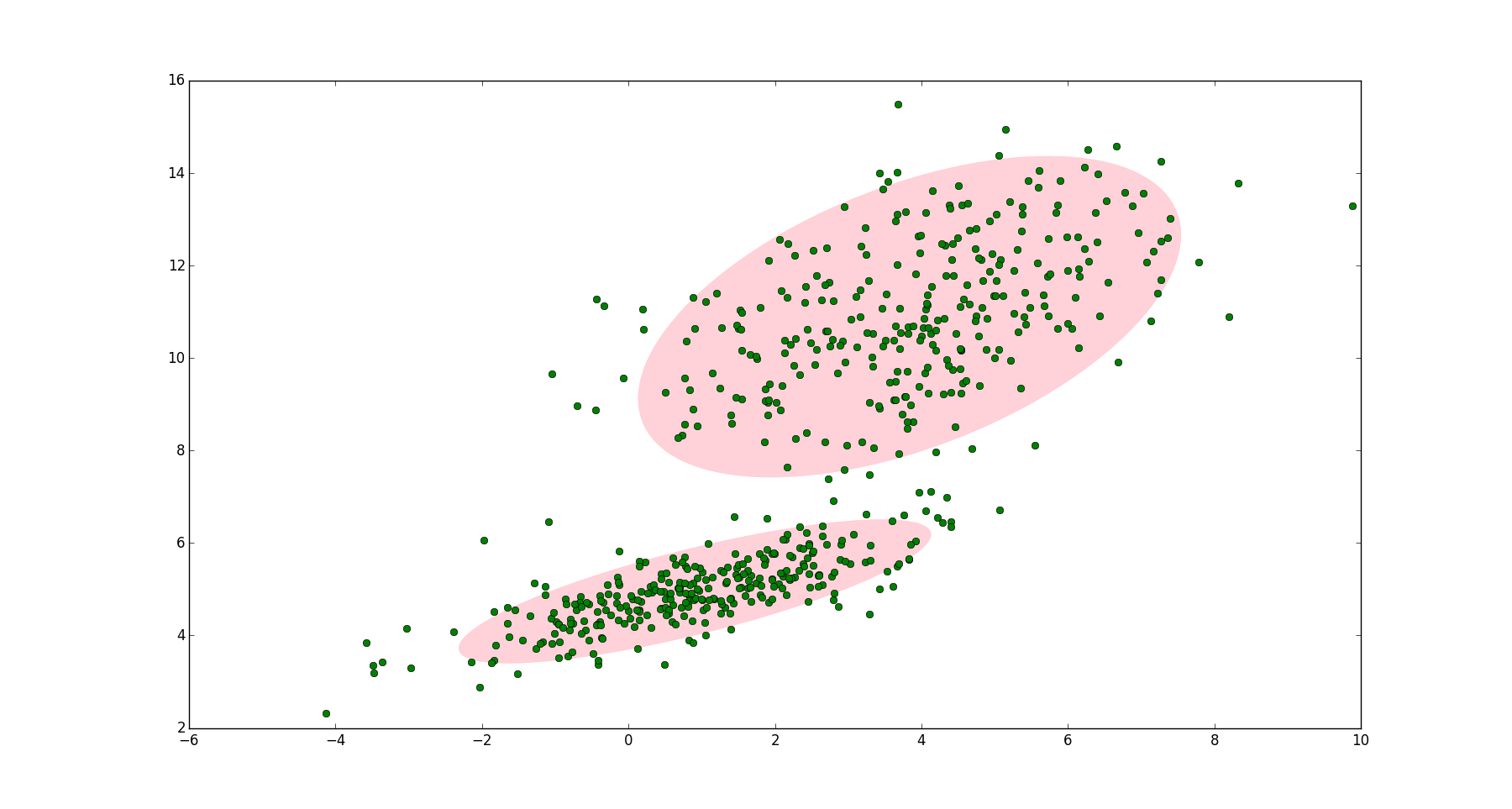• 一个例子高斯混合模型（Gaussian Mixed Model）指的是多个高斯分布函数的线性组合，理论上GMM可以拟合出任意类型的分布，通常用于解决同一集合下的数据包含多个不同的分布的情况（或者是同一类分布但参数不一样，...
一个例子
高斯混合模型（Gaussian Mixed Model）指的是多个高斯分布函数的线性组合，理论上GMM可以拟合出任意类型的分布，通常用于解决同一集合下的数据包含多个不同的分布的情况（或者是同一类分布但参数不一样，或者是不同类型的分布，比如正态分布和伯努利分布）。
如图1，图中的点在我们看来明显分成两个聚类。这两个聚类中的点分别通过两个不同的正态分布随机生成而来。但是如果没有GMM，那么只能用一个的二维高斯分布来描述图1中的数据。图1中的椭圆即为二倍标准差的正态分布椭圆。这显然不太合理，毕竟肉眼一看就觉得应该把它们分成两类。图1
这时候就可以使用GMM了！如图2，数据在平面上的空间分布和图1一样，这时使用两个二维高斯分布来描述图2中的数据，分别记为$\mathcal{N}(\boldsymbol{\mu}_1, \boldsymbol{\Sigma}_1)$和$\mathcal{N}(\boldsymbol{\mu}_2, \boldsymbol{\Sigma}_2)$. 图中的两个椭圆分别是这两个高斯分布的二倍标准差椭圆。可以看到使用两个二维高斯分布来描述图中的数据显然更合理。实际上图中的两个聚类的中的点是通过两个不同的正态分布随机生成而来。如果将两个二维高斯分布$\mathcal{N}(\boldsymbol{\mu_1}, \boldsymbol{\Sigma}_1)$和$\mathcal{N}(\boldsymbol{\mu}_2, \boldsymbol{\Sigma}_2)$合成一个二维的分布，那么就可以用合成后的分布来描述图2中的所有点。最直观的方法就是对这两个二维高斯分布做线性组合，用线性组合后的分布来描述整个集合中的数据。这就是高斯混合模型（GMM）。图2
高斯混合模型（GMM）
设有随机变量$\boldsymbol{X}$，则混合高斯模型可以用下式表示：
$p(\boldsymbol{x}) = \sum_{k=1}^K\pi_k \mathcal{N}(\boldsymbol{x}|\boldsymbol{\mu}_k, \boldsymbol{\Sigma}_k)$
其中$\mathcal{N}(\boldsymbol{x}|\boldsymbol{\mu}_k, \boldsymbol{\Sigma}_k)$称为混合模型中的第$k$个分量（component）。如前面图2中的例子，有两个聚类，可以用两个二维高斯分布来表示，那么分量数$K = 2$. $\pi_k$是混合系数（mixture coefficient），且满足：
$\sum_{k=1}^K\pi_k = 1$
$0 \leq \pi_k \leq 1$
实际上，可以认为$\pi_k$就是每个分量$\mathcal{N}(\boldsymbol{x}|\boldsymbol{\mu}_k, \boldsymbol{\Sigma}_k)$的权重。
GMM的应用
GMM常用于聚类。如果要从 GMM 的分布中随机地取一个点的话，实际上可以分为两步：首先随机地在这 K 个 Component 之中选一个，每个 Component 被选中的概率实际上就是它的系数$\pi_k$ ，选中 Component 之后，再单独地考虑从这个 Component 的分布中选取一个点就可以了──这里已经回到了普通的 Gaussian 分布，转化为已知的问题。
将GMM用于聚类时，假设数据服从混合高斯分布（Mixture Gaussian Distribution），那么只要根据数据推出 GMM 的概率分布来就可以了；然后 GMM 的 K 个 Component 实际上对应$K$个 cluster 。根据数据来推算概率密度通常被称作 density estimation 。特别地，当我已知（或假定）概率密度函数的形式，而要估计其中的参数的过程被称作『参数估计』。
例如图2的例子，很明显有两个聚类，可以定义$K=2$. 那么对应的GMM形式如下：
$p(\boldsymbol{x}) =\pi_1 \mathcal{N}(\boldsymbol{x}|\boldsymbol{\mu}_1, \boldsymbol{\Sigma}_1) + \pi_2 \mathcal{N}(\boldsymbol{x}|\boldsymbol{\mu}_2, \boldsymbol{\Sigma}_2)$
上式中未知的参数有六个：$(\pi_1, \boldsymbol{\mu}_1, \boldsymbol{\Sigma}_1; \pi_2, \boldsymbol{\mu}_2, \boldsymbol{\Sigma}_2)$. 之前提到GMM聚类时分为两步，第一步是随机地在这$K$个分量中选一个，每个分量被选中的概率即为混合系数$\pi_k$. 可以设定$\pi_1 = \pi_2 = 0.5$，表示每个分量被选中的概率是0.5，即从中抽出一个点，这个点属于第一类的概率和第二类的概率各占一半。但实际应用中事先指定$\pi_k$的值是很笨的做法，当问题一般化后，会出现一个问题：当从图2中的集合随机选取一个点，怎么知道这个点是来自$N(\boldsymbol{x}|\boldsymbol{\mu}_1, \boldsymbol{\Sigma}_1)$还是$N(\boldsymbol{x}|\boldsymbol{\mu}_2, \boldsymbol{\Sigma}_2)$呢？换言之怎么根据数据自动确定$\pi_1$和$\pi_2$的值？这就是GMM参数估计的问题。要解决这个问题，可以使用EM算法。通过EM算法，我们可以迭代计算出GMM中的参数：$(\pi_k, \boldsymbol{x}_k, \boldsymbol{\Sigma}_k)$.
GMM参数估计过程
GMM的贝叶斯理解
在介绍GMM参数估计之前，先改写GMM的形式，改写之后的GMM模型可以方便地使用EM估计参数。GMM的原始形式如下：
$p(\boldsymbol{x}) = \sum_{k=1}^K\pi_k \mathcal{N}(\boldsymbol{x}|\boldsymbol{\mu}_k, \boldsymbol{\Sigma}_k) \tag{1}$
前面提到$\pi_k$可以看成是第$k$类被选中的概率。我们引入一个新的$K$维随机变量$\boldsymbol{z}$. $z_k (1 \leq k \leq K)$只能取0或1两个值；$z_k = 1$表示第$k$类被选中的概率，即：$p(z_k = 1) = \pi_k$；如果$z_k = 0$表示第$k$类没有被选中的概率。更数学化一点，$z_k$要满足以下两个条件：
$z_k \in \{0,1\}$
$\sum_K z_k = 1$
例如图2中的例子，有两类，则$\boldsymbol{z}$的维数是2. 如果从第一类中取出一个点，则$\boldsymbol{z} = (1, 0)$；，如果从第二类中取出一个点，则$\boldsymbol{z} = (0, 1)$.
$z_k=1$的概率就是$\pi_k$，假设$z_k$之间是独立同分布的（iid），我们可以写出$\boldsymbol{z}$的联合概率分布形式，就是连乘：
$p(\boldsymbol{z}) = p(z_1) p(z_2)...p(z_K) = \prod_{k=1}^K \pi_k^{z_k} \tag{2}$
因为$z_k$只能取0或1，且$\boldsymbol{z}$中只能有一个$z_k$为1而其它$z_j (j\neq k)$全为0，所以上式是成立的。
图2中的数据可以分为两类，显然，每一類中的数据都是服从正态分布的。这个叙述可以用条件概率来表示：
$p(\boldsymbol{x}|z_k = 1) = \mathcal{N}(\boldsymbol{x}|\boldsymbol{\mu}_k, \boldsymbol{\Sigma}_k)$
即第$k$类中的数据服从正态分布。进而上式有可以写成如下形式：
$p(\boldsymbol{x}| \boldsymbol{z}) = \prod_{k=1}^K \mathcal{N}(\boldsymbol{x}|\boldsymbol{\mu}_k, \boldsymbol{\Sigma}_k)^{z_k} \tag{3}$
上面分别给出了$p(\boldsymbol{z})$和$p(\boldsymbol{x}| \boldsymbol{z})$的形式，根据条件概率公式，可以求出$p(\boldsymbol{x})$的形式：
\begin{aligned} p(\boldsymbol{x}) &= \sum_{\boldsymbol{z}} p(\boldsymbol{z}) p(\boldsymbol{x}| \boldsymbol{z}) \\ &= \sum_{\boldsymbol{z}} \left(\prod_{k=1}^K \pi_k^{z_k} \mathcal{N}(\boldsymbol{x}|\boldsymbol{\mu}_k, \boldsymbol{\Sigma}_k)^{z_k} \right ) \\ &= \sum_{k=1}^K \pi_k \mathcal{N}(\boldsymbol{x}|\boldsymbol{\mu}_k, \boldsymbol{\Sigma}_k) \end{aligned} \tag{4}
（注：上式第二个等号，对$\boldsymbol{z}$求和，实际上就是$\sum_{k=1}^K$。又因为对某个$k$，只要$i \ne k$，则有$z_i = 0$，所以$z_k=0$的项为1，可省略，最终得到第三个等号）
可以看到GMM模型的(1)式与(4)式有一样的形式，且(4)式中引入了一个新的变量$\boldsymbol{z}$，通常称为隐含变量（latent variable）。对于图2中的数据，『隐含』的意义是：我们知道数据可以分成两类，但是随机抽取一个数据点，我们不知道这个数据点属于第一类还是第二类，它的归属我们观察不到，因此引入一个隐含变量$\boldsymbol{z}$来描述这个现象。
注意到在贝叶斯的思想下，$p(\boldsymbol{z})$是先验概率， $p(\boldsymbol{x}| \boldsymbol{z})$是似然概率，很自然我们会想到求出后验概率$p(\boldsymbol{z}| \boldsymbol{x})$：
\begin{aligned} \gamma(z_k) &= p(z_k = 1| \boldsymbol{x}) \\ &= \frac{p(z_k = 1) p(\boldsymbol{x}|z_k = 1)}{p(\boldsymbol{x}, z_k = 1)} \\ &= \frac{p(z_k = 1) p(\boldsymbol{x}|z_k = 1)}{\sum_{j=1}^K p(z_j = 1) p(\boldsymbol{x}|z_j = 1)} \text{ (全概率公式)}\\ &= \frac{\pi_k \mathcal{N}(\boldsymbol{x|\boldsymbol{\mu}_k, \boldsymbol{\Sigma}_k})}{\sum_{j=1}^K \pi_j \mathcal{N}(\boldsymbol{x|\boldsymbol{\mu}_j, \boldsymbol{\Sigma}_j})} \text{ (结合(3)、(4))} \end{aligned} \tag{5}
（第2行，贝叶斯定理。关于这一行的分母，很多人有疑问，应该是$p(\boldsymbol{x}, z_k = 1)$还是$p(\boldsymbol{x})$，按照正常写法，应该是$p(\boldsymbol{x})$。但是为了强调$z_k$的取值，有的书会写成$p(\boldsymbol{x}, z_k = 1)$，比如李航的《统计学习方法》，这里就约定$p(\boldsymbol{x})$与$p(\boldsymbol{x}, z_k = 1)$是等同的）
上式中我们定义符号$\gamma(z_k)$来表示来表示第$k$个分量的后验概率。在贝叶斯的观点下，$\pi_k$可视为$z_k=1$的先验概率。
上述内容改写了GMM的形式，并引入了隐含变量$\boldsymbol{z}$和已知${\boldsymbol{x}}$后的的后验概率$\gamma(z_k)$，这样做是为了方便使用EM算法来估计GMM的参数。
EM算法估计GMM参数
EM算法（Expectation-Maximization algorithm）分两步，第一步先求出要估计参数的粗略值，第二步使用第一步的值最大化似然函数。因此要先求出GMM的似然函数。
假设$\boldsymbol{x} = \{\boldsymbol{x}_1, \boldsymbol{x}_2, ..., \boldsymbol{x}_N\}$，对于图2，$\boldsymbol{x}$是图中所有点（每个点有在二维平面上有两个坐标，是二维向量，因此$\boldsymbol{x}_1, \boldsymbol{x}_2$等都用粗体表示）。GMM的概率模型如(1)式所示。GMM模型中有三个参数需要估计，分别是$\boldsymbol{\pi}$，$\boldsymbol{\mu}$和$\boldsymbol{\Sigma}$. 将(1)式稍微改写一下：
$p(\boldsymbol{x}|\boldsymbol{\pi}, \boldsymbol{\mu}, \boldsymbol{\Sigma}) = \sum_{k=1}^K\pi_k \mathcal{N}(\boldsymbol{x}|\boldsymbol{\mu}_k, \boldsymbol{\Sigma}_k) \tag{6}$
为了估计这三个参数，需要分别求解出这三个参数的最大似然函数。先求解$\mu_k$的最大似然函数。样本符合iid，(6)式所有样本连乘得到最大似然函数，对(6)式取对数得到对数似然函数，然后再对$\boldsymbol{\mu_k}$求导并令导数为0即得到最大似然函数。
$0 = -\sum_{n=1}^N \frac{\pi_k \mathcal{N}(\boldsymbol{x}_n | \boldsymbol{\mu}_k, \boldsymbol{\Sigma}_k)}{\sum_j \pi_j \mathcal{N}(\boldsymbol{x}_n|\boldsymbol{\mu}_j, \boldsymbol{\Sigma}_j)} \boldsymbol{\Sigma}_k^{-1} (\boldsymbol{x}_n - \boldsymbol{\mu}_k) \tag{7}$
注意到上式中分数的一项的形式正好是(5)式后验概率的形式。两边同乘$\boldsymbol{\Sigma}_k$，重新整理可以得到：
$\boldsymbol{\mu}_k = \frac{1}{N_k}\sum_{n=1}^N \gamma(z_{nk}) \boldsymbol{x}_n \tag{8}$
其中：
$N_k = \sum_{n=1}^N \gamma(z_{nk}) \tag{9}$
(8)式和(9)式中，$N$表示点的数量。$\gamma(z_{nk})$表示点$n$（$\boldsymbol{x}_n$）属于聚类$k$的后验概率。则$N_k$可以表示属于第$k$个聚类的点的数量。那么$\boldsymbol{\mu}_k$表示所有点的加权平均，每个点的权值是$\sum_{n=1}^N \gamma(z_{nk})$，跟第$k$个聚类有关。
同理求$\boldsymbol{\Sigma}_k$的最大似然函数，可以得到：
$\boldsymbol{\Sigma}_k = \frac{1}{N_k} \sum_{n=1}^N \gamma(z_{nk}) (x_n - \boldsymbol{\mu}_k)(x_n - \boldsymbol{\mu}_k)^T \tag{10}$
最后剩下$\pi_k$的最大似然函数。注意到$\pi_k$有限制条件$\sum_{k=1}^K\pi_k = 1$，因此我们需要加入拉格朗日算子：
$\ln p(\boldsymbol{x}|\boldsymbol{\pi}, \boldsymbol{\mu}, \boldsymbol{\Sigma}) + \lambda(\sum_{k=1}^K \pi_k -1)$
求上式关于$\pi_k$的最大似然函数，得到：
$0 = \sum_{n=1}^N \frac{\mathcal{N}(\boldsymbol{x}_n | \boldsymbol{\mu}_k, \boldsymbol{\Sigma}_k)}{\sum_j \pi_j \mathcal{N}(\boldsymbol{x}_n|\boldsymbol{\mu}_j, \boldsymbol{\Sigma}_j)} + \lambda \tag{11}$
上式两边同乘$\pi_k$，我们可以做如下推导：
$0 = \sum_{n=1}^N \frac{\pi_k \mathcal{N}(\boldsymbol{x}_n | \boldsymbol{\mu}_k, \boldsymbol{\Sigma}_k)}{\sum_j \pi_j \mathcal{N}(\boldsymbol{x}_n|\boldsymbol{\mu}_j, \boldsymbol{\Sigma}_j)} + \lambda \pi_k \tag{11.1}$
结合公式(5)、(9)，可以将上式改写成：
$0 = N_k + \lambda \pi_k \tag{11.2}$
注意到$\sum_{k=1}^K \pi_k = 1$，上式两边同时对$k$求和。此外$N_k$表示属于第个聚类的点的数量（公式(9)）。对$N_k，$从$k=1$到$k=K$求和后，就是所有点的数量$N$:
$0 = \sum_{k=1}^K N_k + \lambda \sum_{k=1}^K \pi_k \tag{11.3}$
$0 = N + \lambda \tag{11.4}$
从而可得到$\lambda = -N$，带入(11.2)，进而可以得到$\pi_k$更简洁的表达式：
$\pi_k = \frac{N_k}{N} \tag{12}$
EM算法估计GMM参数即最大化(8)，(10)和(12)。需要用到(5)，(8)，(10)和(12)四个公式。我们先指定$\boldsymbol{\pi}$，$\boldsymbol{\mu}$和$\boldsymbol{\Sigma}$的初始值，带入(5)中计算出$\gamma(z_{nk})$，然后再将$\gamma(z_{nk})$带入(8)，(10)和(12)，求得$\pi_k$，$\boldsymbol{\mu}_k$和$\boldsymbol{\Sigma}_k$；接着用求得的$\pi_k$，$\boldsymbol{\mu}_k$和$\boldsymbol{\Sigma}_k$再带入(5)得到新的$\gamma(z_{nk})$，再将更新后的$\gamma(z_{nk})$带入(8)，(10)和(12)，如此往复，直到算法收敛。
EM算法

定义分量数目$K$，对每个分量$k$设置$\pi_k$，$\boldsymbol{\mu}_k$和$\boldsymbol{\Sigma}_k$的初始值，然后计算(6)式的对数似然函数。
E step
根据当前的$\pi_k$、$\boldsymbol{\mu}_k$、$\boldsymbol{\Sigma}_k$计算后验概率$\gamma(z_{nk})$
$\gamma(z_{nk}) = \frac{\pi_k\mathcal{N}(\boldsymbol{x}_n| \boldsymbol{\mu}_n, \boldsymbol{\Sigma}_n)}{\sum_{j=1}^K \pi_j \mathcal{N}(\boldsymbol{x}_n| \boldsymbol{\mu}_j, \boldsymbol{\Sigma}_j)}$
M step
根据E step中计算的$\gamma(z_{nk})$再计算新的$\pi_k$、$\boldsymbol{\mu}_k$、$\boldsymbol{\Sigma}_k$
\begin{aligned} \boldsymbol{\mu}_k^{new} &= \frac{1}{N_k} \sum_{n=1}^N \gamma(z_{nk}) \boldsymbol{x}_n \\ \boldsymbol{\Sigma}_k^{new} &= \frac{1}{N_k} \sum_{n=1}^N \gamma(z_{nk}) (\boldsymbol{x}_n - \boldsymbol{\mu}_k^{new}) (\boldsymbol{x}_n - \boldsymbol{\mu}_k^{new})^T \\ \pi_k^{new} &= \frac{N_k}{N} \end{aligned}
其中：
$N_k = \sum_{n=1}^N \gamma(z_{nk})$
计算(6)式的对数似然函数
$\ln p(\boldsymbol{x}|\boldsymbol{\pi}, \boldsymbol{\mu}, \boldsymbol{\Sigma}) = \sum_{n=1}^N \ln \left\{\sum_{k=1}^K \pi_k \mathcal{N}(\boldsymbol{x}_k| \boldsymbol{\mu}_k, \boldsymbol{\Sigma}_k)\right\}$
检查参数是否收敛或对数似然函数是否收敛，若不收敛，则返回第2步。

GMM聚类的可分性评价
使用GMM得到聚类结果后如何定量评价两个类别的可分性呢？可以通过计算两个或多个类别分布的重叠度来评价模型的可分性。这里介绍一种高斯混合模型的重叠度计算方法：计算高斯混合模型的可分性和重叠度（Overlap Rate, OLR）。
Reference

漫谈 Clustering (3): Gaussian Mixture Model
Draw Gaussian distribution ellipse
Pang-Ning Tan 等, 数据挖掘导论（英文版）, 机械工业出版社, 2010
Christopher M. Bishop etc., Pattern Recognition and Machine Learning, Springer, 2006
李航, 统计学习方法, 清华大学出版社, 2012


展开全文算法
• 高斯混合模型相关资料 包括极大似然估计求解完全推导过程 包括EM求解的完全推导过程 深入浅出 很快就能入门了 高斯混合模型相关资料 包括极大似然估计求解完全推导过程 包括EM求解的完全推导过程 深入浅出 很快就能...
• 高斯混合模型 参数简介II . 高斯混合模型 评分函数III. 似然函数与参数IV . 生成模型法V . 对数似然函数VI . 高斯混合模型方法 步骤 I . 高斯混合模型 参数简介 1 . 模型 与 参数 : 高斯混合模型 概率密度函数 ...
文章目录I . 高斯混合模型 参数简介 ( 参数 )II . 高斯混合模型 评分函数 ( 评价参数 )III. 似然函数与参数IV . 生成模型法V . 对数似然函数VI . 高斯混合模型方法 步骤

I . 高斯混合模型 参数简介 ( 参数 )

1 . 模型 与 参数 : 高斯混合模型 概率密度函数 :

$p(x) = \sum_{i = 1}^k \omega_i g ( x | \mu_i , \Sigma_i )$

模型结构已知 , 即 高斯混合模型 , 需要根据已知的数据样本 , 学习出模型的参数 ;

2 . 高斯混合模型 参数个数 :

① 聚类个数 ( 高斯模型个数 ) : 每个高斯混合模型 都由 $k$ 个高斯模型 ( 组件 ) 线性叠加组成的 ;
② 高斯模型参数 : 每个高斯模型 都有两个参数 , 即 均值 $\mu_i$ , 方差 $\Sigma_i$ ;
③ 样本属于聚类分组概率 ( 系数 ) : 每个高斯模型 还有一个系数参数 , $\omega_i$ 表示该 $x$ 样本由第 $i$ 个 高斯分布 ( 组件 ) 生成的概率 , 也就是 该样本被指派到某个聚类的概率 ;
④ 每个高斯模型相关参数个数 : $k$ 个 高斯模型 , 每个高斯模型有  均值 $\mu_i$ , 方差 $\Sigma_i$ , 生成概率 $\omega_i$ 等 $3$个参数 ;
⑤ 高斯混合模型参数个数 : 整个 高斯混合模型 有 $3 \times k$ 个参数 , $k$ 是聚类分组个数 , 也是高斯模型个数 , 正态分布个数 ;

$\Sigma_i$ 此处方差表示 , 是大写的希腊字母 sigma $\sigma$ , 注意与加和符号 $\sum$ 区分 ;

K-Means 方法中 , 有 $k$ 个参数 , 每个聚类分组 , 只有一个参数 , 即中心点样本参数 ;

II . 高斯混合模型 评分函数 ( 评价参数 )

高斯混合模型 评分函数 :

评价参数 : 为 高斯混合模型 学习训练出的 参数 , 需要 评分函数 来 对参数进行评价 , 评分函数取值 最大 时 , 该 参数是最优参数 ;
似然函数 : 高斯混合模型 中 , 采用似然函数 , 作为评分函数 ;

$E = \prod_{j = 1} ^ n p(x_j)$

$\prod$ 是多个乘积 , 与 $\sum$ 多个加和性质类似 ;
$n$ 表示数据集中样本个数 ;
$x_j$ 表示数据样本对象 , 被聚类的样本点 ;
$p(x_j)$ 表示高斯混合模型中 , $x_j$ 生成的概率 , 也就是 $x_j$ 被分为某个聚类分组的概率 ;

评分函数 ( 似然函数 ) 取值最大时 , 高斯混合模型 的参数最佳 , 使用该 高斯混合模型 , 和对应的 概率 $\omega_i$ , 均值 $\mu_i$ , 方差 $\Sigma_i$ 参数 生成样本数据时 , 与真实的数据集样本 相似的概率最大 ;

III. 似然函数与参数

似然函数 与 参数 :

① 模型生成 样本 概率 : 如果模型参数非常好 , $x_j$ 生成的概率 , 也就是 $x_j$ 属于某个聚类分组的概率是 $100\%$ , 此时 $p(x_j) = 1$ ; 如果 $x_j$ 属于某个聚类分组的概率是 $73\%$ , 此时 $p(x_j) = 0.73$
② 最佳概率 : 极限情况下 , 所有的样本属于某个聚类分组的概率都是 $100\%$ , 每个 $p(x_j) = 1$ , $n$ 个 $p(x_j)$ 相乘也是 $1$ , 该似然函数取值为 $1$ 是理论情况的最佳值 ;
③ 最大似然 : 该似然函数的本质是将每个对象属于某聚类分组的概率相乘 , $E$ 越接近于 $1$ , 参数效果越好 , 此时的 $E$ 称为最大似然 ;

IV . 生成模型法

生成模型法 : 先不看真实数据 , 先用 模型 ( 参数已经训练好 ) 生成数据 , 希望这个模型生成的数据 , 与真实数据是完全相同的 , 如果生成的数据 , 与真实的数据 , 全部完全相同 , 那么分析似然函数的
$E = \prod_{j = 1} ^ n p(x_j)$
乘积 , 其中 $p(x_j) = 1$ , 似然函数 计算过程 就是 $n$ 个 $1$ 相乘 , 其结果是 $1$ ;

但是实际结果肯定不是这样的 , 每个样本的概率 $p(x_j)$ 可能是 $0.9$ , $0.5$ , $0.01$ , 这样 乘起来 值就非常小了 , 参数 和 模型 的 评价结果就是这个最终的乘积越大 , 模型 和 参数 越好 ;

V . 对数似然函数

1 . 似然函数 :  $E = \prod_{j = 1} ^ n p(x_j)$ 是多个 $p(x_j)$ 相乘 , 每个 $p(x_j)$ 值都是小于 $1$ 的数 , 多个小于 $1$ 的数值相乘 , $E$ 的最终计算的值非常小 , 不利于统计计算 ;

2 . 对数运算法则 :

① 两个正数之积的对数 , 等于两个正数对数之和 ;
$log_a ( M \times N ) = log_a M + log_a N$

② 两个正数相除的对数 , 等于两个正数对数相减 ;
$log_a ( \frac{M}{N}) = log_a M- log_a N$

3 . 对数似然函数 : 对上述似然函数取对数 , 就可以将 成绩 $\prod$ 变成 求和 $\sum$ 形式 ;

$\begin{array}{lcl} F &=& logE = log( \prod_{j = 1} ^ n p(x_j) )\\\\ \end{array}$
$F = \sum_{j=1}^n logp(x_j)$
该函数就是对数似然函数 ;

4 . 对数函数 最大值 :

① 无法使用导数 : 对数函数是求和的操作 , 因此该函数无法使用导数方式求最大值 ;
② 迭代求最大值 : 采用逐次迭代 , 的方式求最大值 , 与 K-Means 方法类似 ;
③ 第一次迭代的参数值 : $k$ 个 概率 $\omega_i$ , 均值 $\mu_i$ , 方差 $\Sigma_i$ 参数 , 任意选取 , 随便给出 ;
④ 逐次迭代 : 每次迭代 , 逐步优化这些参数值 , 使 对数似然函数 取值越来越大 ;
⑤ 最佳参数 : 当 对数似然函数 取最大值时 , 此时的参数就是最优参数 ;

VI . 高斯混合模型方法 步骤

1 . 参数初始值设置 :

① 初始状态 ( 第一次迭代 ) : 先给出 $k$ 组参数的初始值 , 每组参数由 概率 $\omega_i$ , 均值 $\mu_i$ , 方差 $\Sigma_i$ 组成 , 参数个数是 $3 \times k$ 个 ;
② 聚类分组个数 : $k$  指的是聚类分组的个数 ;
③ 概率 $\omega_i$ 参数 : 指样本属于某组聚类的概率 ;
④ 均值 $\mu_i$ 参数 : 指的是某组聚类分组的样本 高斯分布 ( 正态分布 ) 的 均值参数 ;
⑤ 方差 $\Sigma_i$ 参数 : 指的是某组聚类分组的样本 高斯分布 ( 正态分布 ) 的 方差参数 ;

2 . 计算概率 :

数据集和分组情况 : 数据集有 $n$ 个对象 , 将这 $n$ 个对象分成 $k$ 个聚类分组 ;
计算的概率 : 这里需要计算每个对象 $x_j \, (1 \leq j \leq n)$ 属于每个聚类 $C_i \, (1 \leq i \leq k)$ 的概率 , 需要计算 $n \times k$ 次概率 ;
概率说明 : $x_j \, (1 \leq j \leq n)$ 属于 聚类 $C_i \, (1 \leq i \leq k)$ 的概率 , 反过来说 , 就是 $x_j$ 样本对象 由 $C_i$ 聚类分组对应的 高斯分布 生成的概率 ;
计算公式 :

$p(x_i \in C_i) = \dfrac{\omega_i g ( x | \mu_i , \Sigma_i )}{ \sum_{i=1}^{k} \, \omega_i g ( x | \mu_i , \Sigma_i ) }$

如果本次迭代是第一次迭代 , 那么上述式子中的参数采用 初始参数设置的值 ;
如果本次迭代不是第一次迭代 , 那么采用上一次迭代得到的参数 值 ;

3 . 计算参数值 : 计算 概率 $\omega_i$ , 均值 $\mu_i$ , 方差 $\Sigma_i$ 参数 ;

① 概率 $\omega_i$ 参数计算公式 : 指样本属于某组聚类的概率 ;

$\omega_i = \frac{n_i}{n}$

② 均值 $\mu_i$ 参数计算公式 : 指的是某组聚类分组的样本 高斯分布 ( 正态分布 ) 的 均值参数 ;

$\mu_i = \frac{1}{n_i} \sum_{j=1} ^n p(x_j \in C_i) x_j$

③ 方差 $\Sigma_i$ 参数计算公式 : 指的是某组聚类分组的样本 高斯分布 ( 正态分布 ) 的 方差参数 ;

$\mu_i = \frac{1}{n_i} \sum_{j=1} ^n p(x_j \in C_i) \,( x_j - \mu_i ) ( x_j - \mu_i ) ^T$

迭代执行 2 , 3 操作 , 直到评分函数达到最大值 ;


展开全文概率密度函数 似然函数 对数似然函数...